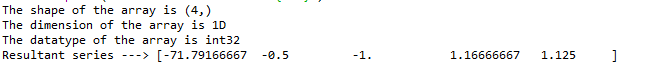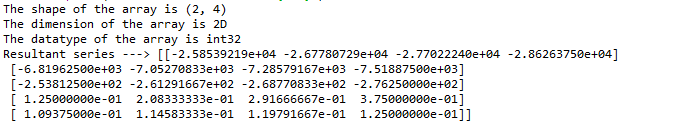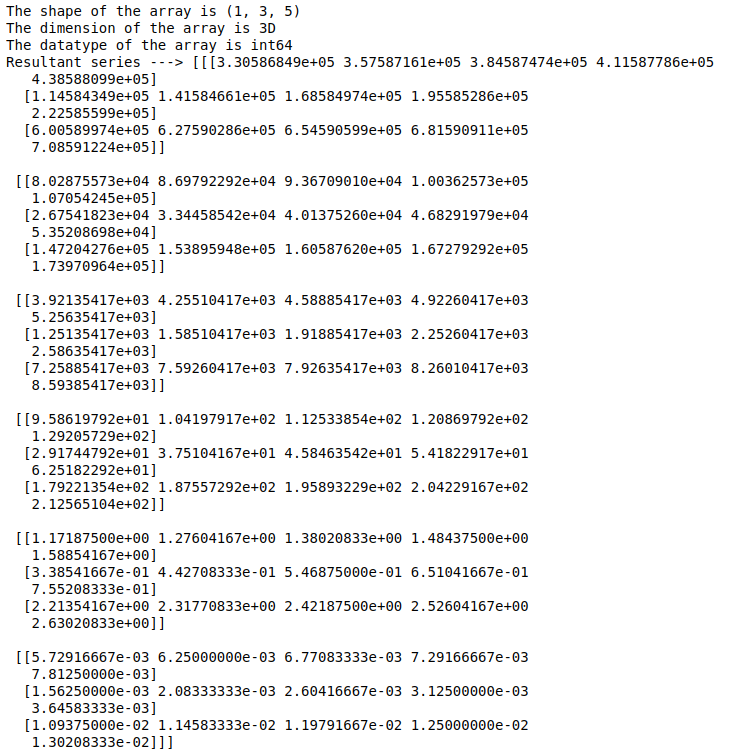Open In App

# Python Program Integrate a Chebyshev Series and Set the Lower Bound of the Integral

The Chebyshev series has polynomials with the largest possible leading coefficient, whose absolute value on the interval [−1, 1] is bounded by 1. They are also the “extremal” polynomials. Chebyshev polynomials are significant in approximation theory because the roots of Tn(x), which are also called Chebyshev nodes, are used as matching points for optimizing polynomial interpolation. The resulting interpolation polynomial minimizes the problem of Runge’s phenomenon and provides an approximation that is close to the best polynomial approximation to a continuous function under the maximum norm, also called the “minimax” criterion.

To perform Chebyshev integration, NumPy provides a function called chebyshev.chebint which can be used to integrate the Chebyshev series.

Syntax: chebyshev.chebint(c, m=1, k=[], lbnd=0, scl=1, axis=0)

Parameters:

• c – Array of Chebyshev series coefficients.
• m – (integer) Order of integration, must be positive
• k –  Integration constant. The value of the first integral at zero is the first value in the list, the value of the second integral at zero is the second value, etc
• lbnd – The lower bound of the integral. (Default: 0)
• scl – Following each integration the result is multiplied by scl before the integration constant is added. (Default: 1)
• axis – Axis over which the integral is taken.

### Example 1:

In the first example. let us consider a 1D array with a first-order integration, 1 as an integration constant, and -2 as the lower bound of the interval. Import the necessary packages as shown and pass the appropriate parameters as shown below.

## Python3

 `import` `numpy as np``from` `numpy.polynomial ``import` `chebyshev`` ` `# co.efficient array``c ``=` `np.array([``3``, ``5``, ``7``, ``9``])`` ` `print``(f``'The shape of the array is {c.shape}'``)``print``(f``'The dimension of the array is {c.ndim}D'``)``print``(f``'The datatype of the array is {c.dtype}'``)`` ` `res ``=` `chebyshev.chebint(c, m``=``1``, k``=``1``, lbnd``=``-``2``)`` ` `# integrated chebyshev series with lower bound of -2``print``(f``'Resultant series ---> {res}'``)`

Output:### Example 2:

In the second example. let us consider a 2D array with a third-order integration, 3 as an integration constant, and -10 as the lower bound of the interval. Import the necessary packages as shown and pass the appropriate parameters as shown below.

## Python3

 `import` `numpy as np``from` `numpy.polynomial ``import` `chebyshev`` ` `# co.efficient array``c ``=` `np.array([[``3``, ``5``, ``7``, ``9``], [``21``, ``22``, ``23``, ``24``]])`` ` `print``(f``'The shape of the array is {c.shape}'``)``print``(f``'The dimension of the array is {c.ndim}D'``)``print``(f``'The datatype of the array is {c.dtype}'``)`` ` `res ``=` `chebyshev.chebint(c, m``=``3``, k``=``3``, lbnd``=``-``10``)`` ` `# integrated chebyshev series with lower bound of -10``print``(f``'Resultant series ---> {res}'`

Output:### Example 3:

In the third example. let us consider a 3D array with a fifth-order integration, 5 as an integration constant, and -20 as the lower bound of the interval. Import the necessary packages as shown and pass the appropriate parameters as shown below.

## Python3

 `import` `numpy as np``from` `numpy.polynomial ``import` `chebyshev`` ` `# co.efficient array``c ``=` `np.array([[[``11``, ``12``, ``13``, ``14``, ``15``],``               ``[``3``, ``4``, ``5``, ``6``, ``7``], ``               ``[``21``, ``22``, ``23``, ``24``, ``25``]]])`` ` `print``(f``'The shape of the array is {c.shape}'``)``print``(f``'The dimension of the array is {c.ndim}D'``)``print``(f``'The datatype of the array is {c.dtype}'``)`` ` `res ``=` `chebyshev.chebint(c, m``=``5``, k``=``5``, lbnd``=``-``20``)`` ` `# integrated chebyshev series with lower bound of -20``print``(f``'Resultant series ---> {res}'``)`

Output: